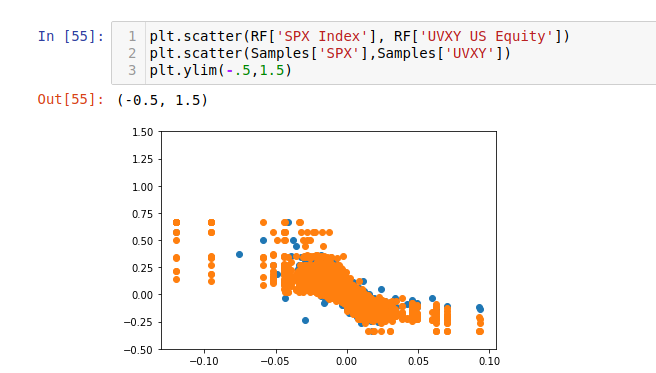Copulas in General

A copula is a mathematical tool for drawing correlated samples from high dimensional distributions, when you know a lot about the one-dimensional marginal probabilities for a few variables but not as much about the correlated samples.

For example, Suppose that you knew the returns of SPX on 4000 days and could construct a reasonable empirical distribution, and likewise the returns of VIX on 4000 days. However only 500 samples were joint samples on the same days. You would like the marginal distributions to have all the quality of the 4000 samples, but also like to train (by maximum likelihood) a correlated model. Copulas are the answer to this problem. They let you use your highly structured uni-variate marginal distributions with the correlation structure of another multivariate distribution.

The main ingredient in a copula is a parent correlated multivariate distribution, for example a multivariate Gaussian. Somewhat confusingly in the mathematical literature, the word copula is associated with the multivariate CDF of this parent distribution. You need to have access to the marginal CDF’s of this multivariate distribution and their inverses, call them: $$\phi_\text{MV}(x),\phi^{-1}_\text{MV}(x)$$

One builds a copula in a few steps:

• Construct your 1d marginal distributions for each series ($$\{ X_i, Y_i \}$$). (for example build the empirical cumulative distribution $$\phi_X(x) = \int_{-\infty}^x \rho_X(x) \approx \sum_i^n (x > X_i)/n$$
• Take the 500 good joint samples you have. Convert each into a sample of the uniform distribution $$\in [0,1]$$ using the CDF of the X,Y distributions: $$\{ \phi_X(X_i), \phi_Y(Y_i) \}$$.
• Now you have samples which are marginally uniform but correlated. Convert them into samples lying in the range of the multivariate distribution by applying the inverse cdf of the multivariate marginal. $$\{ \phi^{-1}_\text{MV}(\phi_X(X_i)), \phi^{-1}_\text{MV}(\phi_Y(Y_i)) \} = \{\tilde{X_i},\tilde{Y_i}\}$$
• Finally use Maximum Likelihood estimation to fix the parameters of the (unit variance) parent multi-variable distribution such that they likelihood of $$\{ \tilde{X_i},\tilde{Y_i} \}$$.

Once such a model is obtained one can draw correlated multivariate samples from the copula by basically following these steps in reverse.

• Generate samples from the correlated multivariate.
• Map them back onto the uniform distribution using $$\phi_\text{MV}$$ for each dimension.
• Use these correlated, but marginally-uniform samples with the inverse CDF of each dimension: $$\phi^{-1}_X, \phi^{-1}_Y \rightarrow \{ X_i , Y_i\}$$

Simple Gaussian Copula’s in Pytorch

Pytorch’s distribution’s module isn’t as well developed as Tensorflow’s or pyro, but I do find it more convenient to use.

The image below compares samples from a copula constructed of SPX and UVXY returns, vs. samples of real returns from each asset.Generalized Gumbel Copula.

A nice feature of maximum likelihood estimation is that when experimenting with different parent multivariates, you can always determine if your fit to the correlation structure of your data is better or not. As far as mathematics goes, copulas are young, and there isn’t a lot of data out there about what’s better or worse. There’s also a dearth of options beyond Gaussian. In my own research, I came across expressions for a copula built on the multivariate Gumbel distribution. Implementing this copula was a lot of work, although for my purposes it didn’t outperform the Gaussian distribution. I’m leaving this here in the hopes it might be of use to someone….